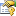﻿ BaseSupportVectorRegression(TModel, TKernel, TInput) PropertiesProperties
NameDescriptionC
Gets or sets the cost values associated with each input vector.Complexity
Complexity (cost) parameter C. Increasing the value of C forces the creation of a more accurate model that may not generalize well. If this value is not set and UseComplexityHeuristic is set to true, the framework will automatically guess a value for C. If this value is manually set to something else, then UseComplexityHeuristic will be automatically disabled and the given value will be used instead.Epsilon
Insensitivity zone ε. Increasing the value of ε can result in fewer support vectors in the created model. Default value is 1e-3.Inputs
Gets or sets the input vectors for training.IsLinear
Gets whether the machine to be learned has a Linear kernel.Kernel
Gets or sets the kernel function use to create a kernel Support Vector Machine. If this property is set, UseKernelEstimation will be set to false.Model
Gets the machine to be taught.Outputs
Gets or sets the output values for each calibration vector.Token
Gets or sets a cancellation token that can be used to stop the learning algorithm while it is running.UseComplexityHeuristic
Gets or sets a value indicating whether the Complexity parameter C should be computed automatically by employing an heuristic rule. Default is false.UseKernelEstimation
Gets or sets whether initial values for some kernel parameters should be estimated from the data, if possible. Default is true.Weights
Gets or sets the individual weight of each sample in the training set. If set to null, all samples will be assumed equal weight. Default is null.
TopSee Also